Visual C++ Object Memory Layout

Published on
17,446 Points
11,146 Views
3 Endorsements
Approved
In our object-oriented world the class is a minimal unit, a brick for constructing our applications. It is an abstraction and we know well how to use it. In well-designed software we are not usually interested in knowing how objects look in memory.
Sometimes the understanding of the memory structure of the C++ class may help in the modern design.
I have to mention that I work with Microsoft Visual Studio and all code posted here was written for this compiler. More then it, I guess that the object memory layout can be different from the one I'm showing here, if you use another compilers. Some "tricks" you will see here are dangerous enough to be used in the production code. It is good to know, nice to understand, but to use such tricks in the real projects... from my point of view it smells very bad.
The following application shows the size of few a trivial objects:
#include <Windows.h>
#include <stdio.h>

class CEmpty
{
};

class C1Int
{
int x;
};

class C2Int
{
int x;
int y;
};

class C3Int
{
int x, y, z;
};

class CInt
{
int x;
public:
CInt() : x(0) {}
};

class CIntDerived: public CInt
{
int y;
public:
CIntDerived() : y (0) {}
};

class CCharArray
{
char x;
};

class CStr
{
char x;
public:
CStr()
{
strcpy(x, "Hello");
}
};

int main()
{
CEmpty empty;

C1Int one;
C2Int two;
C3Int three;

CInt simple;
CIntDerived derived;

CCharArray charArray;
CStr str;

printf("Size of CEmpty object is %d\r\n", sizeof(empty));

printf("Size of C1Int object is %d\r\n", sizeof(one));
printf("Size of C2Int object is %d\r\n", sizeof(two));
printf("Size of C3Int object is %d\r\n", sizeof(three));

printf("Size of CInt object is %d\r\n", sizeof(simple));
printf("Size of CIntDerived object is %d\r\n", sizeof(derived));

printf("Size of CCharArray object is %d\r\n", sizeof(charArray));
printf("Size of CStr object is %d\r\n", sizeof(str));

return 0;
}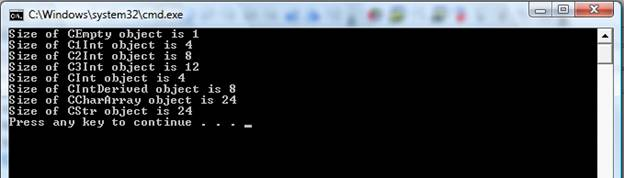As you see the object of the absolutely empty class takes 1 byte in the memory - exactly as it states in the standard - an object of the empty class should occupy 1 byte. Because it is an object and it must have a size. When such empty class is inherited, the standard allows to optimize it away.
Object of the class with only one integer as a member has size 4 bytes. Objects with 2 or 3 integers inside use 8 and 12 bytes accordingly. (Size of the integer type is platform/compiler specific and will vary on diffrent platforms and compilers because the standard does not specify the size of any integral type)
The last declared class with an array as the member-variable has the same size as the array. The class methods do not change the size.
All shown classes have only private members, but all these members can be printed out:
#include <Windows.h>
#include <stdio.h>

class C1Int
{
int x;
public:
C1Int() : x(0) {}
};

class C2Int
{
int x;
int y;
public:
C2Int() : x(1234), y(5678) {};
};

class CStr
{
char x;
public:
CStr()
{
strcpy(x, "Hello");
}
};

int main()
{
C1Int one;
C2Int two;
CStr str;

printf("C1Int::x = %d\r\n", *(int*)&one);

printf("C2Int::x = %d\r\n", *(int*)&two);
printf("C2Int::y = %d\r\n", *((int*)&two + 1));

printf("CStr::x = %s\r\n", (char*)&str);

return 0;
}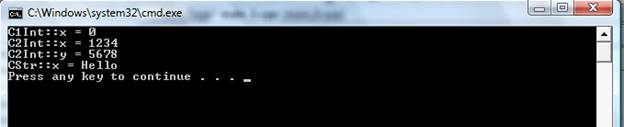An even modify them:
#include <Windows.h>
#include <stdio.h>

class CInts
{
int x;
int y;

public:
CInts() : x(1234), y(5678) {};
void out()
{
printf("CInts::x = %d\r\n", x);
printf("CInts::y = %d\r\n", y);
}
};

int main()
{
CInts one;
one.out();

//the following 2 lines are very dangerous and may cause a crash if you'll
//try to use this style in your projects
*(int*)&one = 111;
*(((int*)&one) + 1) = 222;
one.out();

return 0;
}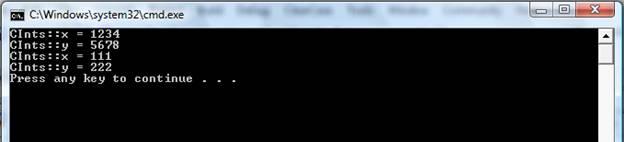In these examples above you see that the object address is the address of the first member-variable of the class. You can see it also in assembler, if you'll stop the debugging of the following example and switch to the assembler window: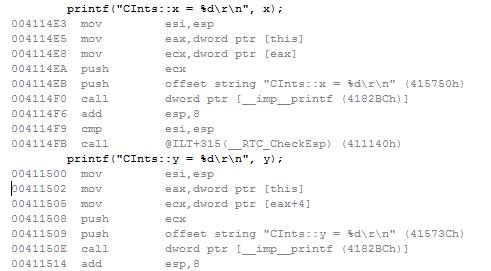The out() method prints both internal class members and you see how both of these variables are pushed into the stack - the address of the first variable is the same as of the class object and the second variable follows in the memory: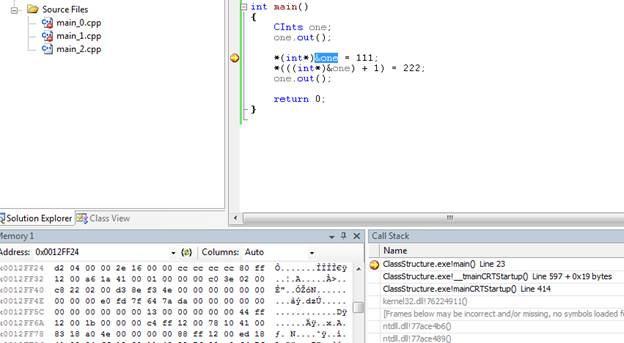What we see in this memory block (CInts object) is just a structure:
struct ints { int x; int y; }
It is also possible to modify the string:
#include <Windows.h>
#include <stdio.h>

class CStr
{
char text;

public:
CStr(char* psz)
{
text = 0;
if (psz != NULL)
strncpy(text, psz, 30);
};
};

int main()
{
CStr str("Hello");
printf("str = %s\r\n", &str);

strcpy((char*)(&str), "bye");
printf("str = %s\r\n", &str);
return 0;
}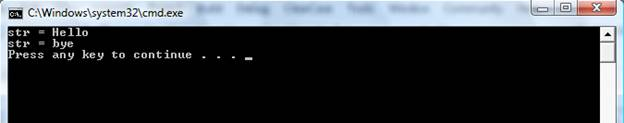All shown is correct untill the virtual functions do not appear on the scene.
The following application shows that even the class that has nothing but only one virtual method takes 4 bytes in the memory.
#include <Windows.h>
#include <stdio.h>

class CEmpty
{
public:
virtual void out()
{
printf("CEmpty\r\n");
}
};

class C1Int
{
int x;
public:
C1Int() : x(0) {}
virtual void out_x()
{
printf("x = %d\r\n", x);
}
};

class C2Int
{
int x, y;
public:
C2Int() : x(0), y(0) {}
virtual void out_x()
{
printf("x = %d\r\n", x);
}
virtual void out_y()
{
printf("y = %d\r\n", y);
}
};

class CIntDerived: public C1Int
{
int y;
public:
CIntDerived() : y (0) {}
virtual void out_y()
{
printf("y = %d\r\n", y);
}
};

int main()
{
CEmpty empty;
C1Int one;
C2Int two;
CIntDerived derived;

printf("Size of CEmpty object is %d\r\n", sizeof(empty));
printf("Size of C1Int object is %d\r\n", sizeof(one));
printf("Size of C2Int object is %d\r\n", sizeof(two));
printf("Size of CIntDerived object is %d\r\n", sizeof(derived));

return 0;
}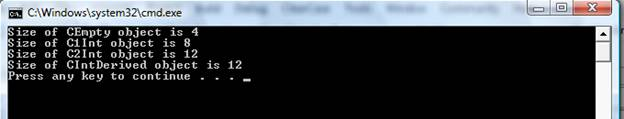Size of CEmpty object (the name is already wrong) is 4 bytes now. Objects of C1Int, C2Int, CIntDerived classes also added 4 bytes. For the Microsoft developers this is a well-known number - size of the integer. It seems like a pointer was added into the memory of these objects.
Let's investigate it in the debug mode. Stop the debugging of the following application and check the object in the memory window (type &str in the edit box there), we will find these 4 bytes - "Hello" is not in the beginning of the object memory. It moved for 4 bytes. And the first 4 bytes are the pointer that we didn't see in the previous examples. It can be only a pointer to the virtual method table.
#include <Windows.h>
#include <stdio.h>

class CStr
{
char text;

public:
CStr(char* psz)
{
text = 0;
if (psz != NULL)
strncpy(text, psz, 30);
}

virtual void out()
{
printf("text = %s\r\n", text);
}
};

int main()
{
CStr str("Hello");
str.out();
return 0;
}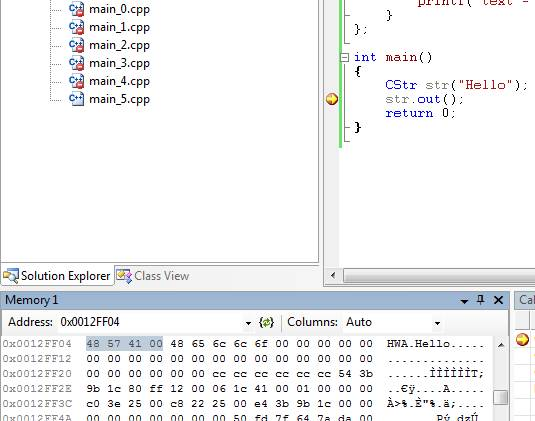This table also can be investigated in the same way as was used for the class internal variables:
#include <stdio.h>

class C1Int
{
int x;

public:
virtual void test()
{
printf("C1Int::test()\r\n");
}
};

class C2Int : public C1Int
{
int y;

public:
virtual void test()
{
printf("C2Int::test()\r\n");
}

virtual void test1()
{
printf("C2Int::test1()\r\n");
}

virtual void test2()
{
printf("C2Int::test2()\r\n");
}
};

int main()
{
C2Int two_int;
printf("size of C2Int object is %d\r\n", sizeof(two_int));
printf("Address of C2Int object is %p\r\n", &two_int);

int* p = (int*)(&two_int);
printf("Address of C2Int pointer is %p\r\n", p);

int p1 = *((int*)(&two_int));
printf("Value of this pointer is %x\r\n", p1);

int* _p1 = (int*)*((int*)(&two_int));
printf("Same..        pointer is %p\r\n", _p1);

int* p2_1 = (int*)*((int*)*(int*)(&two_int));
printf("Value of first entry of C2Int VTable is %p\r\n", p2_1);

int* p2_2 = (int*)*((int*)*(int*)(&two_int) + 1);
printf("Value of second entry of C2Int VTable is %p\r\n", p2_2);

int* p2_3 = (int*)*((int*)*(int*)(&two_int) + 2);
printf("Value of third entry of C2Int VTable is %p\r\n", p2_3);

int* p2_4 = (int*)*((int*)*(int*)(&two_int) + 3);
printf("Value of fourth entry of C2Int VTable is %p\r\n", p2_4);

int* p2_5 = (int*)*((int*)*(int*)(&two_int) + 3);
printf("Value of fifth entry of C2Int VTable is %p\r\n", p2_5);

return 0;
}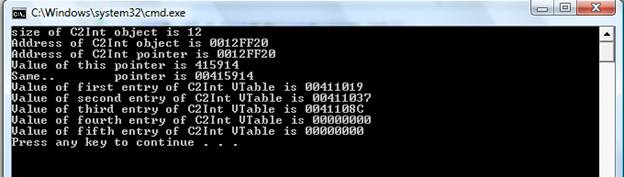The table from the example above has 3 entries, the following entry is NULL. So it is possible to count how many virtual methods are in an object. Thats firstly. Secondly, we can call the methods in a strange way:
#include <stdio.h>

class C1Int
{
public:
virtual void test()
{
printf("C1Int::test()\r\n");
}
};

class C2Int : public C1Int
{
public:
virtual void test()
{
printf("C2Int::test()\r\n");
}

virtual void test1()
{
printf("C2Int::test1()\r\n");
}

virtual void test2()
{
printf("C2Int::test2()\r\n");
}
};

typedef void (* func)();

int main()
{
C2Int two_int;

int* p = (int*)(&two_int);
printf("Address of C2Int pointer is %p\r\n", p);

int p1 = *((int*)(&two_int));
printf("Value of this pointer is %x\r\n", p1);

int* p2_1 = (int*)*((int*)*(int*)(&two_int));
printf("Value of first entry of C2Int VTable is %p\r\n", p2_1);

int* p2_2 = (int*)*((int*)*(int*)(&two_int) + 1);
printf("Value of second entry of C2Int VTable is %p\r\n", p2_2);

int* p2_3 = (int*)*((int*)*(int*)(&two_int) + 2);
printf("Value of third entry of C2Int VTable is %p\r\n", p2_3);

func f1 = (func)p2_1;
f1();

f1 = (func)p2_2;
f1();

f1 = (func)p2_3;
f1();

C1Int one_int;
func f2 = (func)((int*)*((int*)*(int*)(&one_int)));
f2();

return 0;
}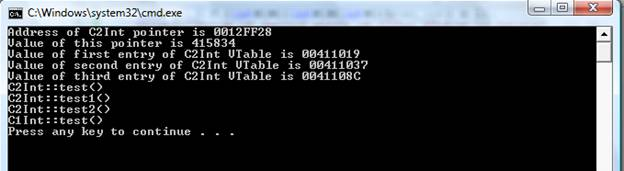Thirdly, with a small trick we can replace a virtual method in an object without permission:
#include <stdio.h>
#include <memory>

class CBase
{
public:
virtual void test()
{
printf("CBase::test()\r\n");
}
};

class CVirtTest
{
public:
virtual void test()
{
printf("CVirtTest::test()\r\n");
}
};

typedef void (* func)();
typedef struct
{
func Entry;
} FUNC_TABLE;

void Trick()
{
printf("Trick()\r\n");
}

int main()
{
CVirtTest test;
CBase* pBase = (CBase*)&test;

FUNC_TABLE funcTable  = { Trick };
FUNC_TABLE* pFuncTable  = &funcTable;
//dangerous code, don't use it in your projects.
memcpy(&test, &pFuncTable, sizeof(long));

pBase->test();
return 0;
}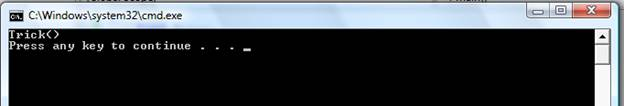So I can say that for the Microsoft compiler, an object of a C++ class in the memory is a continuous memory buffer that contains class member-variables and, optionally, a pointer to to the virtual method table. This virtual method table is presented if the class contains at least one virtual method.
I think the following pseudocode illustrates everyhting I was talking about:
An object of the class declared as:
class ClassStructure
{
int x;
int y;
public:
virtual void nothing() {};
}
in the memory will look as the following structure:
struct ClassStructure
{
void* m_pVptr;
int x;
int y;
}
The article does not contain any example or an explanation about the multiple inheritence.  Other interesting things about the object memory layout are not covered also. You can find more information here:
Wikipedia: C++ classes.
Memory Layout for Multiple and Virtual Inheritance.
or in this book:
Steve Oualline. Practical C++ Programming, Second Edition.
3